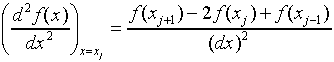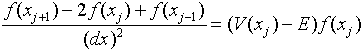## Physics 252 Homework

Due April 16, 1999

### Solving Schrödinger's Equation for the Finite Square Well

The object of this homework assignment is to find out how to use Excel spreadsheets to solve the time-independent Schrödinger equation, and thereby gain insight into how the wave function behaves for various potentials.

We choose units so that, and denote the wave function by f(x) or just f.

We have:To solve this using the spreadsheet, it is necessary to discretize the derivative. We replace the continuous variable x by a sequence of equally spaced points dx apart, such as 0, dx, 2dx, 3dx, … . Here dx is of course finite, we should really call it delta_x.

We approximate the second derivative by:so the differential equation is replaced by the difference equation:Notice that from this equation, if we know f(xj), f(xj-1) and V(xj) we can find f(xj+1). Of course, we do know V(xj), this is the potential we are trying to solve for. We can also specify E - the energy of the particle (although we shall find that some values of E do not correspond to stationary states with finite wave function everywhere).

A second-order differential equation like this needs two boundary conditions for the solution to be defined. We can specify the initial value of f and its derivative df/dx. But this is just equivalent to giving the first two members of the discrete series, f(x0) and f(x1), say. We can then use the difference equation to find f(x2), then use it again to find f(x3) and so on. This is what the spreadsheet does for us - we find f(xj+1) row by row using the difference equation above written:### Constructing the Spreadsheet

Give it a title, write your name, and a brief account of what it will do at the top (this can be revised later!).

Assign squares for the input: the energy E, and the values f(0) and f'(0), say, if we start at the origin (the spreadsheet will translate these into f(x0) and f(x1)). The other important physical parameters are the depth of the well D, and the width of the well (twice the distance from the origin to the wall) is W. Specify also the step size dx. This will have to be adjusted on changing E usually. (I put the values of E, f(0), f'(0), dx, D and W into B15, 16, 17, 18, 19 and 20 respectively.) Also, it's a good idea to put some rather arbitrary numbers into these cells, this will make it easier to see if your formulas are working later. Try, for example, E = 1, f(0) = 1, f'(0) = 0, dx = 0.01, D = 5 and W = 3.14159, this unarbitrary last choice gives simple eigenvalues in the limit of a very deep well, where the problem becomes that of a particle confined in a box, as discussed in the last lecture.

Insert Names: it's good practice to use Insert/Name/Define. to name the square where you type your energy value E, the well depth D, the step value square dx, and the width of the well W. Just give them the names E, D, etc. You can then use these names when you write formulas in other squares, and it's easier to follow what you're doing. NOTE: Before you name cell B15 E, type E= into the cell to its immediate left, A15. Then, when you click on B15 and click Insert/Name/Define, Excel will suggest the name E. For f(0), it will suggest f_0, and then for f'(0) it will suggest f__0. These are OK, because you can't have parentheses in a legal Excel name, apparently.

Assign three columns (I used D, E, F) to position x, V(x) - E, and f(x) respectively. Label the columns in row 15, typing position x into D15, V(x) - E into E15 and wavefunction f(x) into F15. Now you are ready to put in some numbers. Type 0 into D16, then the formula =D16+dx into D17 (you must have already typed some numerical value into the square named dx). If you see the appropriate value coming up in D17, select (highlight) D17, click copy, and drag downwards about 600 rows (this will go fast if you drag hard). (Actually, you don't need to click Copy -- when you click on the cell, the black line around its border has an extra little square at the bottom right-hand corner. Place the cursor on that little square so that the cursor itself becomes a black +sign (as opposed to the usual Excel cursor of a white + sign with a black shadowed border). You can click and hold on that and drag it down to copy.) When all the cells you're copying to are black, just click to unhighlight the whole column.

We are going to solve the differential equation numerically by integrating out from the origin. To solve it for the square well potential, we take the origin to be in the middle of the well. This is because the square well is symmetrical about its middle, and we shall show in the lectures that for such a potential all the solutions of the wave equation can be taken to be either symmetrical or antisymmetrical about the middle. Therefore, we only have to find the solution in the right-hand half. The symmetrical solutions will have zero slope at the origin, the antisymmetrical solutions will equal zero at the origin.

Since the wavefunction is related to the probability of finding the particle somewhere, to describe a particle in a well the wave function must be small when we are far from the well. Putting an arbitrary value of the energy in the equation, you are going to find that generally the wave function increases rapidly at large distances. That means a particle cannot be trapped in the well at that energy. You have to adjust E until the wave function decreases at large distances. This energy corresponds to a bound state in the well.

To describe the square well potential, I used the Excel SIGN function, which is equal to one for positive numbers, -1 for negative numbers. Therefore, my first entry in the V(x) - E column is =D*0.5* (1+SIGN(D16-0.5*W))-E (this assumes you have assigned names, and there are some values in the named cells).

The function column: my column F. The first entry is just f(x0) = f(0), so enter = f_0. The second entry f(x1) = f(0) + f'(0)dx, so put =f_0 + dx*f__0.

The third entry in the function column is the first to use the formula: in square F18, enter:

=(2 + dx*dx*E18)*F17 - F16

Now copy this formula down 600 rows. It will solve Schrödinger's equation for you to a good approximation.

Make a graph: plot the wave function and V(x)-E as functions of x on the same graph. (Highlight cells D15 through F600 then click on Chartwizard. Choose XY Scatter, then the top right-hand choice (no markers, smooth lines). Next, put in the title "Square Well" and label the x-axis position x. Click the Gridlines tag and get rid of the horizontal gridlines.

If your graph is mostly flat, then takes off towards the end, it's because the automatic scale assigned to the y-axis is being determined by the divergence of the wavefunction at large x. This is not the interesting part of the wavefunction. You need to rescale. Right click on the y axis, click Format Axis, click the scale tag, and adjust the scale: say, max and min of 5, units of 1, and click the autoscale to "off" -- that is, get rid of the checkmarks in the autoscale column. To see what is happening to the wavefunction, you don't want it too flattened by choosing the wrong scale, nor do you want substantial parts of it disappearing off your graph. The thing to do is to experiment with changing f(0) until you get the best view. For this square well example, it works best when f(0) is near the maximum value of your y-axis, but this isn't always the best choice, as we shall see. (There is actually a correct value of f(0) for bound states, found from the normalization of the wavefunction, but that can be fixed at the end. Meanwhile, our adjusting of f(0) is just magnifying or demagnifying the wavefunction for a better view.)

### Exercises

1. Fix the width of the well by putting W = 3.14159, and put D=1. Take dx = 0.02. (But you can vary it as you wish.) Take your wavefunction to equal one at the origin, and have zero slope. Vary the energy near 0.35 to 0.37 to look for the bound state. Print your best wavefunction. Adjust the value of f(0) to give the clearest picture.

NOTE: In this and the following exercises, it's worth trying different values of dx. If you take dx too small, the wavefunction to the far right may not be going gradually to zero, as it would for a bound state, but may merely be passing through zero at the last point on your graph. This is not what you want, because it will then begin diverging to infinity just beyond your graph, and so be a poor approximation to the true wavefunction. On the other hand, if you take dx too large, your square well is crowded at the left-hand end of your graph, the integration is less accurate, and this combined with the large distance outside the well where you require the wavefunction to be very small makes it very difficult to pin down the wavefunction over the whole range of the graph. And, in fact, for finding the energy of the bound state, it's not necessary -- any wavefunction with a visible "flat" part in practice gives the energy to an excellent approximation.

2. Now take a deep well, say D=20. Find the energy (around 0.8) of the bound state. Now show that there is another bound state at about nine times that energy. Compare the two wavefunctions.

3. Now look for an odd bound state, f(-x) = -f(x). (Hint: there's one at about four times the energy of the lowest bound state found above.)

4. Look at wave functions for the energy greater than the well depth. Comment on how the phase and wavelength change at the boundary.

5. Make a copy of your spreadsheet and replace the potential with a simple harmonic oscillator potential, equal to x2. Find the energies of the first four states (two even, two odd), sketch the wave functions. Now look at a very high energy state (many wiggles) and see if you can relate the probability distribution to a classical simple harmonic oscillator.